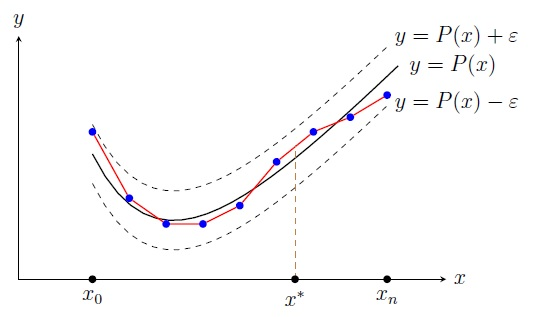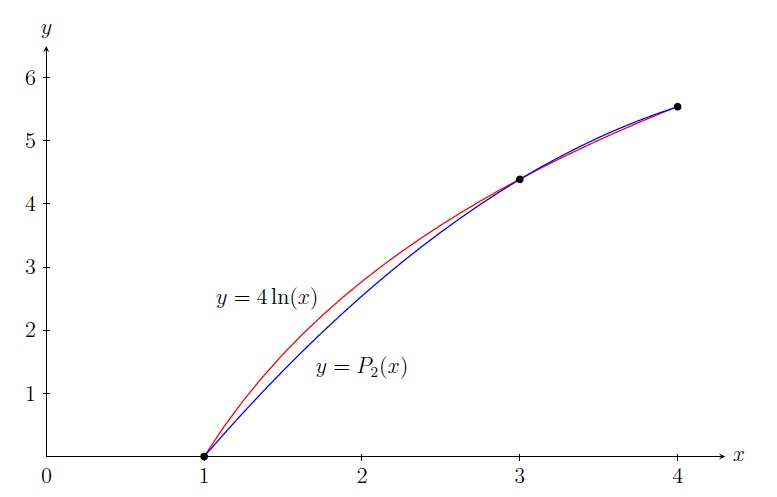Numerical Analysis Home

## Lagrange Polynomials

Suppose we have a set of data in which for each $$x_i$$ you have $$y_i$$: $\begin{array}{|l|l|} \hline x_0 & y_0\\ \hline x_1 & y_1\\ \hline x_2 & y_2\\ \hline x_3 & y_3\\ \hline \vdots & \vdots\\ \hline x_n & y_n\\ \hline \end{array}$ So there is an unknown function $$f$$ for which $$f(x_i)=y_i,\; i=0,1,\ldots,n$$. With this data we would like to predict $$f(x^*)$$ for a given $$x^*\in [x_0,x_n]$$ where $$x^*\neq x_i,\; i=0,1,\ldots,n$$. This method of finding an untabulated data from a given table of data is called interpolation.

How do we find or approximate the unknown function $$f$$? One easy answer is to get a piece-wise linear function $$f^*$$ such that $$f^*(x)=y_i+(x-x_i)\frac{y_i-y_{i-1}}{x_i-x_{i-1}}$$ for all $$x\in [x_{i-1},x_i]$$. But this is too naive because it assumes the functional values are changing at a constant rate $$\frac{y_i-y_{i-1}}{x_i-x_{i-1}}$$ on the entire interval $$[x_{i-1},x_i]$$.There are multiple techniques of approximating $$f$$. We will mainly focus on approximating $$f$$ by a polynomial $$P_n$$ of degree $$n$$, called the interpolating polynomial and the method is called the polynomial interpolation. The polynomial interpolation is suggested by the following theorem:

Theorem.[Weierstrass Approximation Theorem] For a given continuous function $$f$$ on $$[a, b]$$ and a small positive number $$\varepsilon$$, there exists a polynomial $$P$$ such that $|f(x) - P(x)|<\varepsilon,$ $$\text{ i.e., } P(x)-\varepsilon< f(x)< P(x)+\varepsilon,$$ for all $$x$$ in $$[a, b]$$.

How to find such a polynomial $$P$$? What is the maximum error in approximating $$f(x^*)$$ by $$P(x^*)$$?

For two distinct points $$(x_0,y_0)$$ and $$\;(x_1,y_1)$$, there is a unique polynomial $$P_1$$ of degree 1 such that $$P_1(x_0)=y_0$$ and $$P_1(x_1)=y_1$$. It can be verified that $P_1(x)=y_0+\frac{y_1-y_0}{x_1-x_0}(x-x_0) =y_0\frac{x-x_1}{x_0-x_1}+y_1\frac{x-x_0}{x_1-x_0},$ whose graph is the straight line joining $$(x_0,y_0)$$ and $$(x_1,y_1)$$. We can extend this idea to $$n+1$$ distinct points:

Theorem. Suppose $$(x_0,y_0),\;(x_1,y_1),\ldots,(x_n,y_n)$$ are $$n+1$$ distinct points where $$x_0,x_1,\ldots,x_n$$ are distinct and $$f$$ is a function such that $$f(x_i)=y_i,\;i=0,1,\ldots,n$$. Then there is a unique polynomial $$P_n$$ of degree at most $$n$$ such that $$P_n(x_i)=f(x_i),\;i=0,1,\ldots,n$$.

Proof.(Sketch) Consider a polynomial $$P_n(x)=a_0+a_1x+a_2x^2+\cdots+a_nx^n$$ for which $$P_n(x_i)=f(x_i)=y_i,\;i=0,1,\ldots,n$$. It gives us a system of $$n+1$$ equations in $$n+1$$ variables $$a_0,a_1,\ldots,a_n$$: $a_0+a_1x_i+a_2x_i^2+\cdots+a_nx_i^n=y_i,\;i=0,1,\ldots,n.$ Its matrix form is $$A\overrightarrow{x}=\overrightarrow{b}$$ where $$\overrightarrow{x}=[a_0,\ldots,a_n]^T$$, $$\overrightarrow{b}=[y_0,\ldots,y_n]^T$$, and $$A$$ is the Vandermonde matrix $A=\left[\begin{array}{cccc} 1 & x_0 & \cdots & x_0^n\\ 1 & x_1 & \cdots & x_1^n\\ \vdots & \vdots & \ddots & \vdots\\ 1 & x_n & \cdots & x_n^n \end{array} \right].$ The determinant $$\det(A)=\displaystyle\prod_{0\leq i< j\leq n}(x_j-x_i)\neq 0$$ as $$x_0,x_1,\ldots,x_n$$ are distinct. So $$A$$ is invertible and we have a unique solution $$[a_0,\ldots,a_n]^T=\overrightarrow{x}=A^{-1}\overrightarrow{b}$$ giving a unique polynomial $$P_n$$ of degree at most $$n.\;$$ ◼

Note that there are infinitely many polynomials $$P$$ of degree more than $$n$$ for which $$P(x_i)=f(x_i)=y_i,\;i=0,1,\ldots,n$$. One construction of the polynomial $$P_n$$ of degree at most $$n$$ such that $$P_n(x_i)=f(x_i)=y_i,\;i=0,1,\ldots,n$$ is given by Joseph Lagrange:

Lagrange Polynomial: $$P_n(x)=y_0L_0(x)+y_1L_1(x)+\cdots+y_nL_n(x),$$ where $$L_i$$ is given by $L_i(x)=\displaystyle\prod_{\substack{j=0\\j\neq i}}^n\frac{(x-x_j)}{(x_i-x_j)} =\frac{(x-x_0)\cdots(x-x_{i-1})(x-x_{i+1})\cdots(x-x_n)}{(x_i-x_0)\cdots(x_i-x_{i-1})(x_i-x_{i+1})\cdots(x_i-x_n)}.$ Note that $$L_i(x_i)=1$$ and $$L_i(x_j)=0$$ for all $$j\neq i$$. Thus $$P_n(x_i)=y_i=f(x_i),\;i=0,1,\ldots,n$$.

Example. Approximate $$f(2)$$ by constructing the Lagrange polynomial $$P_2$$ of degree $$2$$ from the following data: $\begin{array}{|r|r|} \hline x & f(x)\\ \hline 1 & 0\\ \hline 3 & 4.39\\ \hline 4 & 5.54\\ \hline \end{array}$
Solution. $$P_2$$ is given by $$P_2(x)=y_0L_0(x)+y_1L_1(x)+y_2L_2(x),$$ where \begin{align*} L_0(x)&=\frac{(x-3)(x-4)}{(1-3)(1-4)}=\frac{(x-3)(x-4)}{6},\\ L_1(x)&=\frac{(x-1)(x-4)}{(3-1)(3-4)}=\frac{(x-1)(x-4)}{-2},\\ L_2(x)&=\frac{(x-1)(x-3)}{(4-1)(4-3)}=\frac{(x-1)(x-3)}{3}. \end{align*} Thus \begin{align*} P_2(x)&=y_0L_0(x)+y_1L_1(x)+y_2L_2(x)\\ &=0\frac{(x-3)(x-4)}{6}+4.39\frac{(x-1)(x-4)}{-2}+5.54\frac{(x-1)(x-3)}{3}\\ &=4.39\frac{(x-1)(x-4)}{-2}+5.54\frac{(x-1)(x-3)}{3}. \end{align*} Thus $$f(2)\approx P_2(2)=2.54.$$The preceding example has the table for $$f(x)=4\ln x$$.

Maximum Error: If a function $$f$$ that has continuous $$f^{(n+1)}$$ on $$[x_0,x_n]$$ is interpolated by the Lagrange polynomial $$P_n$$ using $$n+1$$ points $$(x_0,y_0),\;(x_1,y_1),\ldots,(x_n,y_n)$$, then the error is given by the following for each $$x\in [x_0,x_n]$$: $f(x)-P_n(x)=\frac{f^{(n+1)}(\xi)}{(n+1)!}(x-x_0)(x-x_1)\cdots(x-x_n),$ where $$\xi\in (x_0,x_n)$$ depends on $$x$$.

Proof. (Sketch) If $$x=x_i,\;i=0,1,\ldots,n$$, then $f(x_i)-P_n(x_i)=0=\frac{f^{(n+1)}(\xi)}{(n+1)!}(x_i-x_0)(x_i-x_1)\cdots(x_i-x_n).$ For a fixed $$x\neq x_i,\;i=0,1,\ldots,n$$, define a function $$g$$ on $$[x_0,x_n]$$ by $g(t)=f(t)-P_n(t)-[f(x)-P_n(x)]\displaystyle\prod_{j=0}^n\frac{(t-x_j)}{(x-x_j)}.$ Verify that $$g^{(n+1)}$$ is continuous on $$[x_0,x_n]$$ and $$g$$ is zero at $$x,x_0,x_1,\ldots,x_n$$. Then by the Generalized Rolle's Theorem, $$g^{(n+1)}(\xi)=0$$ for some $$\xi\in (x_0,x_n)$$ which implies (steps skipped) $f^{(n+1)}(\xi)-0-[f(x)-P_n(x)]\frac{(n+1)!}{\displaystyle\prod_{j=0}^n(x-x_j)}=0.$ Now solving for $$f(x)$$, we get $f(x)-P_n(x)=\frac{f^{(n+1)}(\xi)}{(n+1)!}(x-x_0)(x-x_1)\cdots(x-x_n).\; ◼$

So the maximum error is given by $\boxed{|f(x)-P_n(x)|\leq \frac{MK}{(n+1)!} }$ where $$M=\displaystyle\max_{x\in [x_0,x_n]} |f^{(n+1)}(x)|$$ and $$K=\displaystyle\max_{x\in [x_0,x_n]} |(x-x_0)(x-x_1)\cdots(x-x_n)|$$.

This error bound does not have a practical application as $$f$$ is unknown. But it shows that the error decreases when we take more points $$n$$ (most of the times!).

Note that if $$f$$ is a polynomial of degree at most $$n$$, then $$f^{(n+1)}=0$$ and consequently $$M=0$$ giving no error.

Example. Find the maximum error in approximating $$f(x)=4\ln x$$ on $$[1,4]$$ by the Lagrange polynomial $$P_2$$ using points $$x=1,3,4$$.

Solution. Since $$|f'''(x)|=8/x^3$$ is decreasing on $$[1,4]$$, $$M=\displaystyle\max_{x\in [1,4]} |f'''(x)|=|f'''(1)|=8.$$

Now we find extremum values of $$g(x)=(x-1)(x-3)(x-4)=x^3-8x^2+19x-12$$. $g'(x)=3x^2-16x+19=0 \implies x=(8\pm \sqrt{7})/3.$ Note that $$(8\pm \sqrt{7})/3\in [1,4]$$ and \begin{align*} g((8+ \sqrt{7})/3) &=(20-14\sqrt{7})/27 \\ g((8- \sqrt{7})/3) &=(20+14\sqrt{7})/27 \\ g(1) &=0\\ g(4) &=0. \end{align*} Since $$|g((8- \sqrt{7})/3)|> |g((8+ \sqrt{7})/3)|$$, $K=\displaystyle\max_{x\in [1,4]} |(x-1)(x-3)(x-4)|=(20+14\sqrt{7})/27.$ Thus the maximum error is $\frac{MK}{(n+1)!}=\frac{8\cdot (20+14\sqrt{7})/27}{3!}=2.81.$ The last example has the table for $$f(x)=4\ln x$$. So $$f(2)=4\ln 2=2.77$$ and the absolute error is $$|P_2(2)-f(2)|=|2.54-2.77|=0.23$$. But approximating $$f(x)$$ by $$P_2(x)$$ for any $$x\in [1,4]$$ will have the maximum error $$2.81$$.

Note that another construction of the unique polynomial $$P_n$$ of degree at most $$n$$ such that $$P_n(x_i)=f(x_i),\;i=0,1,\ldots,n$$ is given by Issac Newton: $P_n(x)=a_0+a_1(x-x_0)+a_2(x-x_0)(x-x_1)+\cdots+a_n(x-x_0)\cdots(x-x_{n-1}),$ where $$a_i,\;i=0,\ldots,n$$ are found by Divided Differences.

Last edited